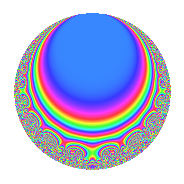# Properties

 Label 15.5.fLevel 15 Weight 5 Character orbit f Rep. character $$\chi_{15}(7,\cdot)$$ Character field $$\Q(\zeta_{4})$$ Dimension 8 Newforms 1 Sturm bound 10 Trace bound 0

# Related objects

## Defining parameters

 Level: $$N$$ = $$15 = 3 \cdot 5$$ Weight: $$k$$ = $$5$$ Character orbit: $$[\chi]$$ = 15.f (of order $$4$$ and degree $$2$$) Character conductor: $$\operatorname{cond}(\chi)$$ = $$5$$ Character field: $$\Q(i)$$ Newforms: $$1$$ Sturm bound: $$10$$ Trace bound: $$0$$

## Dimensions

The following table gives the dimensions of various subspaces of $$M_{5}(15, [\chi])$$.

Total New Old
Modular forms 20 8 12
Cusp forms 12 8 4
Eisenstein series 8 0 8

## Trace form

 $$8q$$ $$\mathstrut -\mathstrut 84q^{5}$$ $$\mathstrut +\mathstrut 36q^{6}$$ $$\mathstrut +\mathstrut 20q^{7}$$ $$\mathstrut +\mathstrut 180q^{8}$$ $$\mathstrut +\mathstrut O(q^{10})$$ $$8q$$ $$\mathstrut -\mathstrut 84q^{5}$$ $$\mathstrut +\mathstrut 36q^{6}$$ $$\mathstrut +\mathstrut 20q^{7}$$ $$\mathstrut +\mathstrut 180q^{8}$$ $$\mathstrut +\mathstrut 104q^{10}$$ $$\mathstrut -\mathstrut 288q^{11}$$ $$\mathstrut -\mathstrut 360q^{12}$$ $$\mathstrut -\mathstrut 340q^{13}$$ $$\mathstrut +\mathstrut 144q^{15}$$ $$\mathstrut +\mathstrut 620q^{16}$$ $$\mathstrut +\mathstrut 900q^{17}$$ $$\mathstrut +\mathstrut 564q^{20}$$ $$\mathstrut +\mathstrut 792q^{21}$$ $$\mathstrut -\mathstrut 1100q^{22}$$ $$\mathstrut -\mathstrut 1560q^{23}$$ $$\mathstrut -\mathstrut 1204q^{25}$$ $$\mathstrut -\mathstrut 3024q^{26}$$ $$\mathstrut +\mathstrut 3580q^{28}$$ $$\mathstrut -\mathstrut 2664q^{30}$$ $$\mathstrut -\mathstrut 512q^{31}$$ $$\mathstrut +\mathstrut 4980q^{32}$$ $$\mathstrut +\mathstrut 2700q^{33}$$ $$\mathstrut +\mathstrut 6600q^{35}$$ $$\mathstrut +\mathstrut 2484q^{36}$$ $$\mathstrut -\mathstrut 3820q^{37}$$ $$\mathstrut -\mathstrut 7680q^{38}$$ $$\mathstrut -\mathstrut 2952q^{40}$$ $$\mathstrut -\mathstrut 2712q^{41}$$ $$\mathstrut -\mathstrut 7380q^{42}$$ $$\mathstrut -\mathstrut 1240q^{43}$$ $$\mathstrut -\mathstrut 1944q^{45}$$ $$\mathstrut +\mathstrut 13528q^{46}$$ $$\mathstrut +\mathstrut 4800q^{47}$$ $$\mathstrut +\mathstrut 3600q^{48}$$ $$\mathstrut +\mathstrut 3744q^{50}$$ $$\mathstrut +\mathstrut 6264q^{51}$$ $$\mathstrut -\mathstrut 1240q^{52}$$ $$\mathstrut +\mathstrut 1020q^{53}$$ $$\mathstrut -\mathstrut 3644q^{55}$$ $$\mathstrut -\mathstrut 30720q^{56}$$ $$\mathstrut -\mathstrut 5400q^{57}$$ $$\mathstrut +\mathstrut 2340q^{58}$$ $$\mathstrut -\mathstrut 1044q^{60}$$ $$\mathstrut -\mathstrut 4760q^{61}$$ $$\mathstrut +\mathstrut 28680q^{62}$$ $$\mathstrut +\mathstrut 540q^{63}$$ $$\mathstrut -\mathstrut 1212q^{65}$$ $$\mathstrut +\mathstrut 10008q^{66}$$ $$\mathstrut -\mathstrut 8920q^{67}$$ $$\mathstrut -\mathstrut 1920q^{68}$$ $$\mathstrut +\mathstrut 7380q^{70}$$ $$\mathstrut +\mathstrut 7536q^{71}$$ $$\mathstrut -\mathstrut 4860q^{72}$$ $$\mathstrut +\mathstrut 11600q^{73}$$ $$\mathstrut -\mathstrut 5976q^{75}$$ $$\mathstrut +\mathstrut 4344q^{76}$$ $$\mathstrut -\mathstrut 360q^{77}$$ $$\mathstrut -\mathstrut 4680q^{78}$$ $$\mathstrut +\mathstrut 10644q^{80}$$ $$\mathstrut -\mathstrut 5832q^{81}$$ $$\mathstrut -\mathstrut 27200q^{82}$$ $$\mathstrut -\mathstrut 32400q^{83}$$ $$\mathstrut -\mathstrut 15628q^{85}$$ $$\mathstrut +\mathstrut 14592q^{86}$$ $$\mathstrut +\mathstrut 10620q^{87}$$ $$\mathstrut -\mathstrut 14340q^{88}$$ $$\mathstrut +\mathstrut 8964q^{90}$$ $$\mathstrut +\mathstrut 16528q^{91}$$ $$\mathstrut -\mathstrut 31800q^{92}$$ $$\mathstrut +\mathstrut 14040q^{93}$$ $$\mathstrut +\mathstrut 18864q^{95}$$ $$\mathstrut -\mathstrut 4068q^{96}$$ $$\mathstrut +\mathstrut 58640q^{97}$$ $$\mathstrut +\mathstrut 46440q^{98}$$ $$\mathstrut +\mathstrut O(q^{100})$$

## Decomposition of $$S_{5}^{\mathrm{new}}(15, [\chi])$$ into irreducible Hecke orbits

Label Dim. $$A$$ Field CM Traces $q$-expansion
$$a_2$$ $$a_3$$ $$a_5$$ $$a_7$$
15.5.f.a $$8$$ $$1.551$$ $$\mathbb{Q}[x]/(x^{8} - \cdots)$$ None $$0$$ $$0$$ $$-84$$ $$20$$ $$q+\beta _{1}q^{2}+\beta _{5}q^{3}+(-\beta _{1}-12\beta _{2}-2\beta _{3}+\cdots)q^{4}+\cdots$$

## Decomposition of $$S_{5}^{\mathrm{old}}(15, [\chi])$$ into lower level spaces

$$S_{5}^{\mathrm{old}}(15, [\chi]) \cong$$ $$S_{5}^{\mathrm{new}}(5, [\chi])$$$$^{\oplus 2}$$